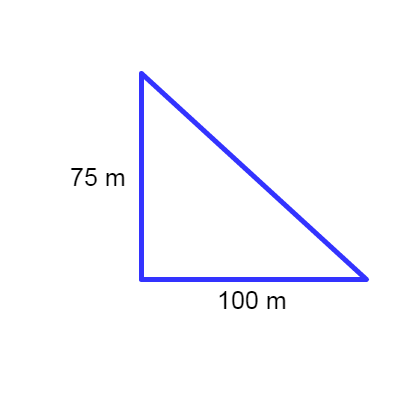# A boat is 100 m from the bottom of a 75 m high cliff. Calculate the angle of elevation from the...

## Question:

A boat is 100 m from the bottom of a 75 m high cliff. Calculate the angle of elevation from the boat to the top of the cliff.

## Tangent Function:

This function depends on the legs of a rectangular triangle, and is useful for finding information about the triangle such as internal angles. The tangent function is given by: {eq}\tan\theta=\frac{opposite}{adjacent} {/eq}{eq}\begin{align*} \tan\theta&=\frac{75\:m}{100\:m} \\ \tan\theta&=\frac{3}{4} \\ \theta&=\tan^{-1}\left(\frac{3}{4}\right) \\ \theta&=36.87^{\circ} \end{align*} {/eq}